Basic Formulas: Operation on Large Numbers

# Basic Formulas: Operation on Large Numbers - Notes | Study Mathematics for Class 5 - Class 5

 1 Crore+ students have signed up on EduRev. Have you?

Measurements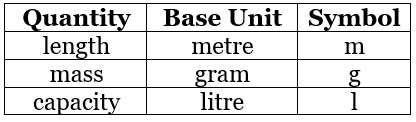• If we consider metre, litre, and gram as units of measurement, the higher units are obtained by adding the prefixes deca (meaning ten), hecto (meaning hundred) and kilo (meaning thousand).
• The lower units are obtained by adding the prefixes deci (meaning tenth), centi (meaning hundredth), milli (meaning thousandth)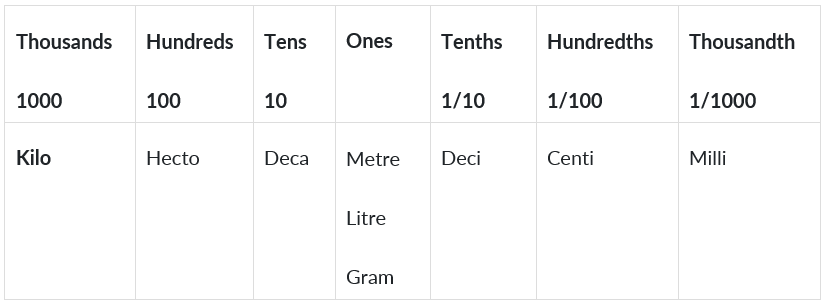Measures of length

• Length is the longest extent of anything as measured from end to end.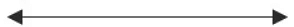• In the above example length is
• used to measure how long the line is.
• 10 millimetres (mm) = 1 centimetre
• 10 centimetres (cm) = 1 decimetre
• 10 decimetres (dm) = 1 metre
• 10 metres (m) = 1 decametre
• 10 decametres (dam) = 1 hectometre
• 10 hectometres (hm) = 1 kilometre (km)

Measures of mass

• Mass is the measure of amount of matter in an object.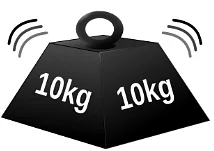• 10 milligrams (mg) = 1 centigram
• 10 centigrams (cg) = 1 decigram
• 10 decigrams (dg) = 1 gram
• 10 grams (g) = 1 decagram
• 10 decagrams (dag) = 1 hectogram
• 10 hectograms (hg) = 1 kilogram (kg)

Measures of capacity
Capacity is the amount a container can hold

• 10 millilitres (ml) = 1 centilitre
• 10 centilitres (cl) = 1 decilitre
• 10 decilitres (dl) = 1 litre
• 10 litres (l) = 1 decalitre
• 10 decalitres (dal) = 1 hectolitre
• 10 hectolitres (hl) = 1 kilolitre (kl)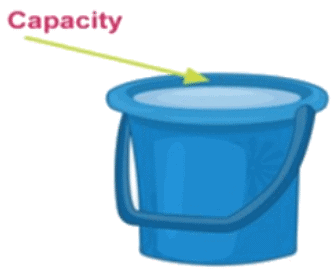Measures of volume
Volume is the measure of the space taken up by something.

• 1000 cubic millimetres (mm3) = 1 cubic centimetre
• 1000 cubic centimetres (cm3) = 1 cubic decimetre
• 1000 cubic decimetres (dm3) = 1 cubic metre
• 1000 cubic metres (m3) = 1 cubic decametre
• 1000 cubic decametres (dam3) = 1 cubic hectometre
• 1000 cubic hectometres (hm3) = 1 cubic kilometre (km3)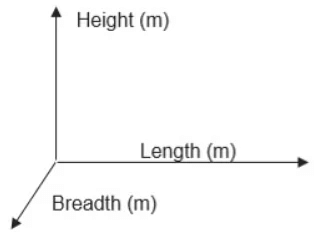Volume = Length (m) X Breadth (m) X Height (m)
Unit of volume is cubic length, i.e., cubic metre (m3), cubic centimetre (cm3), etc

Example 1: Express 1357.912 metres in higher units and lower units.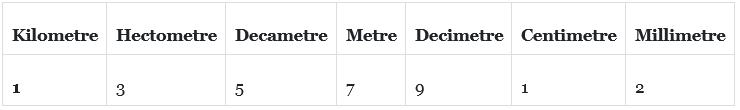1357.912 metre
= 135.7912 decametres
= 13.57912 hectometres
= 1.357912 kilometres
= 13579.12 decimetres
= 135791.2 centimetres
= 1357912 millimetres

Note: Capacity and Volume are different. For example. the capacity of a cylinder is 33 litres while its volume is πR2H (R = radius, H = Height of the cylinder). That is. the amount of gas inside the cylinder is 33 litres but the volume occupied by the Cylinder is πR2H.

Example 2: Express 2 g 3 dg 4cg 5mg in terms of milligrams.

2g = 2000mg
3dg = 300mg
4cg = 40mg
2g 3dg 4cd 5mg = (2000 + 300 + 40 + 5) mg =2345mg

• Add the numbers in each position, if a carry is generated then add the carry with the digits in the next position.

Example 1: Add 2l 3dl 4cl 5ml & 6l 6dl 7cl 8ml

2l 3dl 4cl 5ml + 6l 6dl 7cl 8ml 9l 0dl 2cl 3ml [Ans]

Example 2: Express the above result in terms of ml.

2345ml + 6678ml 9023ml

Note: We cannot add or subtract two different quantities. For example, length (metre) cannot be added with mass (g), mass (g) cannot be added with volume (m3), and volume (m3) cannot be added with length (m)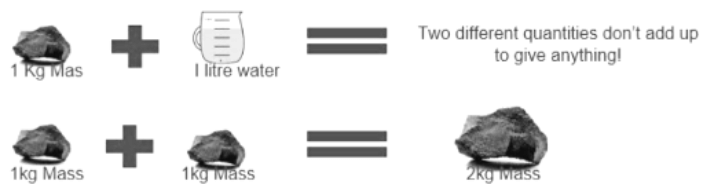• Subtract the number in each position, if a borrow is taken then add it to the next position where subtraction will take place.

Example 1: Subtract 3m3 4dm3 1cm5mm3 from 4m3 3dm3 2cm3 1mm3 .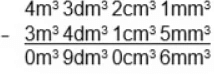Example 2: Express the above in mm3 and write the result.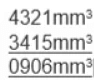Measurements: Multiplication and Division

• When a quantity is multiplied by a number, we are actually adding the same quantity a repeated number of times.
For example,
When 15kg is multiplied
by 3, we are actually adding
the amount 15kg three times.
• When a quantity is divided by a number, we are actually splitting the quantity.
For example,
6 litres of oil is
to be divided into
3 separate containers.
Here, we are dividing 6 by 3.
which is equal to 2. Thus, each
container would contain 2 litres of oil.
• Two different quantities can be multiplied or divided. When we do so, we get a new quantity.
For example,
consider two quantities
mass (kg) and volume (m3).
When we divide these two, we
Kg/m3 get Kg/mis the unit of another quantity called density.

Example 1: Divide 5kg 3m3 by 5m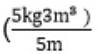= 1 kg 3m2

Temperature

• Temperature is the measure of the hotness or coldness of a substance. A thermometer is an instrument which is used to measure temperature.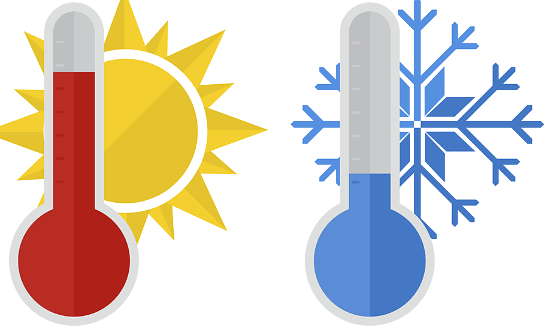Hot and cold
• Temperature is measured in three scales: Celsius, Fahrenheit, and Kelvin.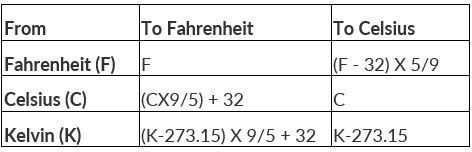• Celsius a measurementof temperature on a standard in which 0° is the temperature at which water freezes, and 100° the temperature at which it boils.
Celsius scale is also

Fahrenheit Scale: A temperature scale on which water freezes at 32 degrees Fahrenheit and boils at 212 degrees Fahrenheit.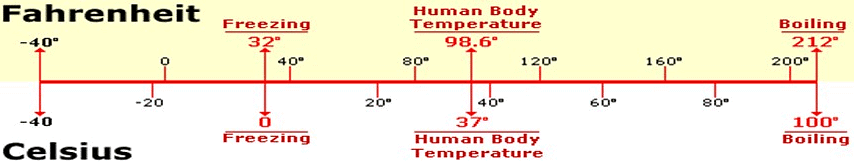Kelvin Scale: A temperature scale that defines absolute zero as 0 degrees. Water freezes at 273 degrees and boils at 373 degrees at this temperature. All objects emit thermal energy or heat unless they have a temperature of absolute zero.

Example 1: Convert 123 degrees Fahrenheit into Celsius and Kelvin.

Celsius = (F-32) x 5/9 = (123-32) x 5/9 =  50.55 degree Celsius Kelvin = Celsius + 273 = 50.55 + 273 = 323.55 K

Example 2: Convert 45 Celsius into Fahrenheit and kelvin.

Fahrenheit = (Celsius x 9/5) + 32 = (45 x 9/5) + 32 = 113 degrees Fahrenheit Kelvin = Celsius + Fahrenheit = 45+273 = 318 K

The document Basic Formulas: Operation on Large Numbers - Notes | Study Mathematics for Class 5 - Class 5 is a part of the Class 5 Course Mathematics for Class 5.
All you need of Class 5 at this link: Class 5

## Mathematics for Class 5

12 videos|55 docs|35 tests
 Use Code STAYHOME200 and get INR 200 additional OFF

## Mathematics for Class 5

12 videos|55 docs|35 tests

Track your progress, build streaks, highlight & save important lessons and more!

,

,

,

,

,

,

,

,

,

,

,

,

,

,

,

,

,

,

,

,

,

;